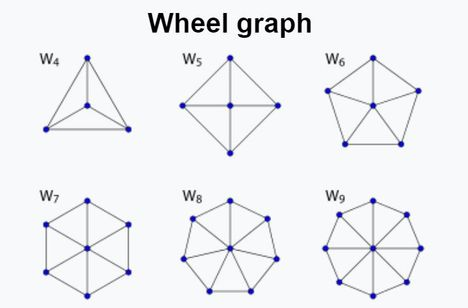# Practice on Toph

Participate in exhilarating programming contests, solve unique algorithm and data structure challenges and be a part of an awesome community.

# Wheel Graph

By fsshakkhor · Limits 1s, 512 MB

Today we will learn about a special type of graph called Wheel Graph. In the mathematical discipline of graph theory, a wheel graph is a graph formed by connecting a single vertex to all vertices of a cycle. A cycle in a graph is a non-empty trail in which the only repeated vertices are the first and last vertices. A wheel graph containing N vertices is denoted by Wn.(Image source: Wikipedia)

You will be given a wheel graph containing N vertices. Suppose all the edges of the wheel graph has unit weight. Let D(A,B) be the shortest distance from vertex A to vertex B.

I want to know the sum of all D(A,B) where A (1 ≤ A ≤ N), B (1 ≤ B ≤ N) and A≠B.

## Input

The first line contains an integer T (1 ≤ T ≤ 10000), denoting the number of test cases.
Each of the next T lines contain an integer N (4 ≤ N ≤ 10000).

## Output

For each test case, you have to print the answer in a single line.

## Sample

InputOutput
2
4
5

12
24


•Math

### Statistics

92% Solution Ratio

s_dasailorEarliest, Dec '19

s_dasailorLightest, 131 kB

bokaifShortest, 48B

### Submit